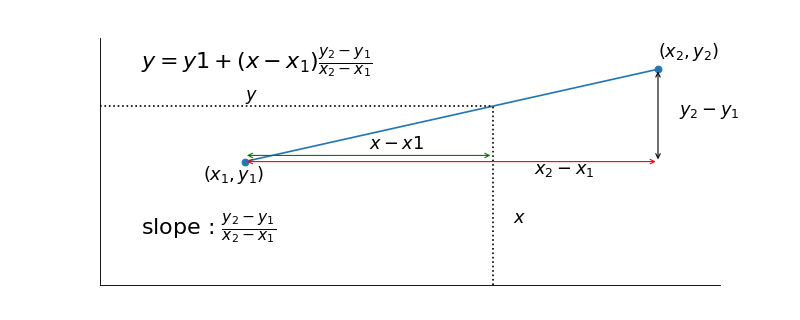$$\newcommand{L}{\| #1 \|}\newcommand{VL}{\L{ \vec{#1} }}\newcommand{R}{\operatorname{Re}\,(#1)}\newcommand{I}{\operatorname{Im}\, (#1)}$$

# Linear interpolation¶

Let us say that we have two known points $$x_1, y_1$$ and $$x_2, y_2$$.

Now we want to estimate what $$y$$ value we would get for some $$x$$ value that is between $$x_1$$ and $$x_2$$. Call this $$y$$ value estimate — an interpolated value.

Two simple methods for choosing $$y$$ come to mind. The first is see whether $$x$$ is closer to $$x_1$$ or to $$x_2$$. If $$x$$ is closer to $$x_1$$ then we use $$y_1$$ as the estimate, otherwise we use $$y_2$$. This is called nearest neighbor interpolation.

The second is to draw a straight line between $$x_1, y_1$$ and $$x_2, y_2$$. We look to see the $$y$$ value on the line for our chosen $$x$$. This is linear interpolation.

It is possible to show that the formula of the line between $$x_1, y_1$$ and $$x_2, y_2$$ is:

$y = y_1 + (x-x_1)\frac{y_2-y_1}{x_2-x_1}$

(png, hires.png, pdf)